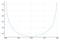# When points become a misleading abstraction

## From divergences in log-likelihood calculations to the heart of renormalization theory

A few days ago I elaborated here on how points, due to their infinite precision nature, are no good as foundations for understanding space. As with all idealizations, they can be useful or not, depending on the situation. Here I’d like to present a simple, practical situation I recently run into in which points fail. I will then use it to briefly illustrate the idea behind renormalization in theoretical physics.The density of a beta distribution with parameters α=0.1 and β=0.1.

Consider a grey-scale image. We want to model its pixels with a random variable. Since they take values in the interval [0,1], it makes sense to model them using a beta distribution, say X ∼ β(0.1, 0.1). Note that the probability density function diverges at both 0 and 1; nevertheless, Prob(X=0) = Prob(X=1) = 0, so, from a theoretical perspective, this divergence should cause no annoyances. The situation is different in practice: since numbers can only be specified to finite precision, sampling X one is bound to get the values 0 and 1 quite often — enough so as to be a source of `Inf`’s and `Nan`’s that would render the model unusable, depending on the application. Let us get an idea of how bad this can be:

`julia> β = Beta(.1,.1)Beta{Float64}(α=0.1, β=0.1)julia> x = [rand(β) for _ in 1:1000];julia> l = logpdf.(β,x);julia> extrema(l)(-1.7336960997885573, Inf)julia> sum(l .== Inf)21`

Since there’s usually much more than 1000 pixels in an image, we should expect troubles trying to make, say, a maximum likelihood estimation of the parameters α and β via gradient descent.

Of course, one can try replacing 0 and 1 with, say, 0.00001 and 0.99999 and just keep going; but that’d be ugly and boring. We’ve got a divergence here, one that should somehow not be there, and that’s a precious opportunity to understand the shortcomings of our standard, point-based conceptual toolkit. How would a principled solution to this problem look like?

Let us begin by recalling that the probability density function f(x) should basically be understood as the limit of the quotient Prob(XU)/Vol(U) as Vol(U) decreases to 0. Here, U is a region containing x and Vol(U) is actually a length, because we are in a one-dimensional case. There is no reason for this limit to exist, let alone be finite; however, Prob(XU) must be a well-defined number between 0 and 1, provided U itself is reasonable enough (the technical term is measurable). Thus, it suffices to revert to using reasonable U’s instead of points.

This, upon careful thought, is actually more or less what we’ve being doing all along, but in a sleight-handed manner. In fact, since floating point numbers have only finite precision, whenever we say X = 0, that can only mean X U with U = [0,ϵ) or any other fundamentally equivalent conventional choice, where ϵ is the smallest representable floating point number greater than 0, namely

`julia> eps(0.)5.0e-324`

Thus, whenever we run into the problematic f(0) = ∞, that’s because we should have actually used the perfectly finite

`julia> cdf(β,eps(0.))/eps(0.)4.795180605114553e290`

The same is true for values other than 0, namely f(x) should systematically be replaced by `cdf(β,x+eps(x))/eps(x)`, but of course that would only make a difference in exceptional cases like the above.

In brief, divergences disappear if one recalls that the probability density function is an idealization built on the notion of infinitesimal point: correctly taking into account the inherent granularity of any actual computation means using finite difference quotients of the cumulative density function instead. Which brings to the front another interesting problem that was previously hidden under the rug of potential “numerical errors”: is it possible that the parameters that we infer for our model depend on the granularity of the computation? Otherwise said: if, for instance, we use Float32’s instead of Float64’s, should we really expect to get roughly the same parameters? In a simple model like this one, I would certainly think so, but the possibility of it being otherwise must not be dismissed as a numerical artifact. It is precisely what happens in quantum field theory! There, the parameters of the model depend on the discretization of space-time (here, we have discretized the grey-scale color space), in such a bad way indeed that they diverge in the limit of infinite precision. Accepting that there is no theoretical problem with such a possibility is called renormalization theory and took literally decades to be developed! One might wonder: had we been more aware of the pitfalls of basing our understanding on points rather than regions, having thus developed from the beginning foundations for applied mathematics not so heavily biased towards classical real analysis, would it have taken so long?

Mathematical physicist turned AI researcher. Knows something about deep learning and the mathematics of quantum fields. Currently works at Ennova Research.

## More from Rodrigo Vargas

Mathematical physicist turned AI researcher. Knows something about deep learning and the mathematics of quantum fields. Currently works at Ennova Research.